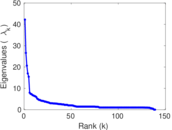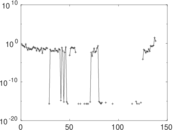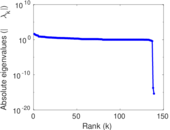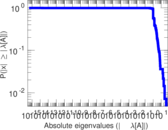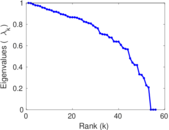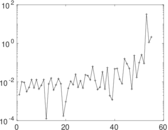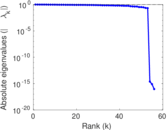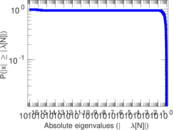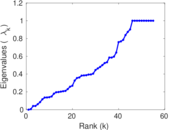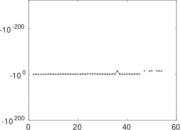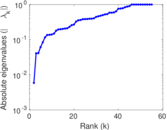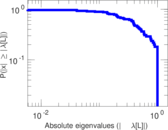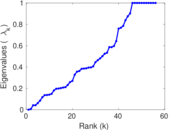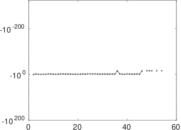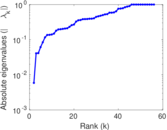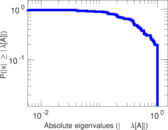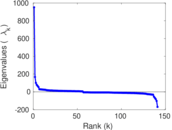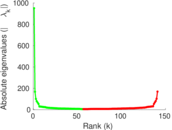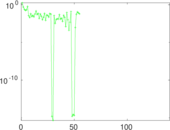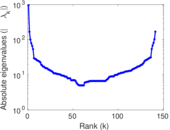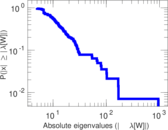# Wikibooks edits (tg)

This is the bipartite edit network of the Tajik Wikibooks. It contains users and pages from the Tajik Wikibooks, connected by edit events. Each edge represents an edit. The dataset includes the timestamp of each edit.

 Code `btg` Internal name `edit-tgwikibooks` Name Wikibooks edits (tg) Data source http://dumps.wikimedia.org/ AvailabilityDataset is available for download Consistency checkDataset passed all tests Category Authorship network Dataset timestamp 2017-10-20 Node meaning User, article Edge meaning Edit Network formatBipartite, undirected Edge typeUnweighted, multiple edges Temporal dataEdges are annotated with timestamps

## Statistics

 Size n = 960 Left size n1 = 141 Right size n2 = 819 Volume m = 1,308 Unique edge count m̿ = 933 Wedge count s = 38,672 Claw count z = 1,846,652 Cross count x = 71,875,138 Square count q = 139 4-Tour count T4 = 157,682 Maximum degree dmax = 220 Maximum left degree d1max = 220 Maximum right degree d2max = 42 Average degree d = 2.725 00 Average left degree d1 = 9.276 60 Average right degree d2 = 1.597 07 Fill p = 0.008 079 39 Average edge multiplicity m̃ = 1.401 93 Size of LCC N = 708 Diameter δ = 16 50-Percentile effective diameter δ0.5 = 3.979 18 90-Percentile effective diameter δ0.9 = 7.768 50 Median distance δM = 4 Mean distance δm = 5.194 83 Gini coefficient G = 0.620 273 Balanced inequality ratio P = 0.259 174 Left balanced inequality ratio P1 = 0.174 312 Right balanced inequality ratio P2 = 0.379 205 Relative edge distribution entropy Her = 0.828 605 Power law exponent γ = 6.053 46 Tail power law exponent γt = 2.831 00 Tail power law exponent with p γ3 = 2.831 00 p-value p = 0.002 000 00 Left tail power law exponent with p γ3,1 = 1.841 00 Left p-value p1 = 0.071 000 0 Right tail power law exponent with p γ3,2 = 3.481 00 Right p-value p2 = 0.001 000 00 Degree assortativity ρ = −0.183 650 Degree assortativity p-value pρ = 1.602 53 × 10−8 Spectral norm α = 42.201 9 Algebraic connectivity a = 0.005 875 15 Spectral separation |λ1[A] / λ2[A]| = 1.586 03 Controllability C = 667 Relative controllability Cr = 0.708 820

## Plots

### Fruchterman–Reingold graph drawing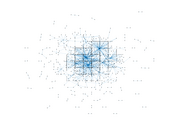### Degree distribution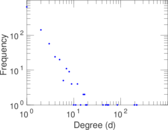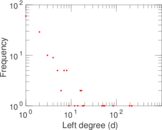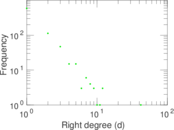### Cumulative degree distribution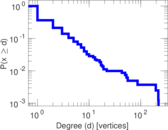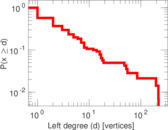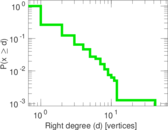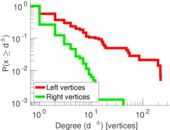### Lorenz curve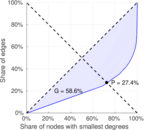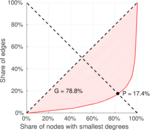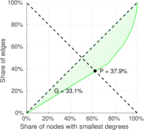### Spectral distribution of the adjacency matrix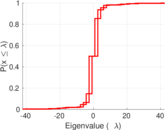### Spectral distribution of the normalized adjacency matrix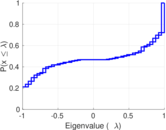### Spectral distribution of the Laplacian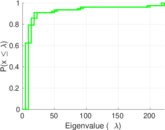### Spectral graph drawing based on the adjacency matrix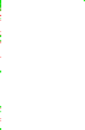### Spectral graph drawing based on the Laplacian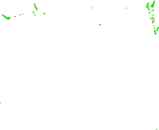### Spectral graph drawing based on the normalized adjacency matrix### Degree assortativity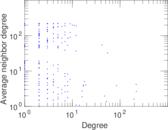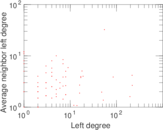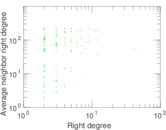### Zipf plot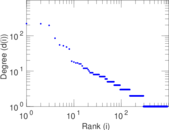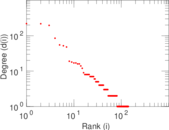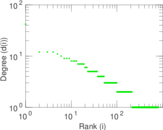### Hop distribution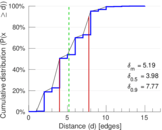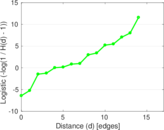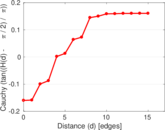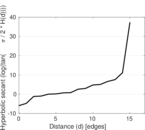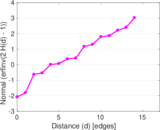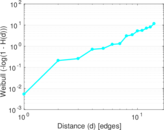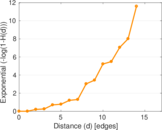### Double Laplacian graph drawing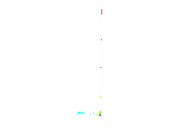### Delaunay graph drawing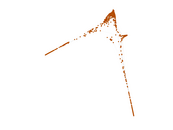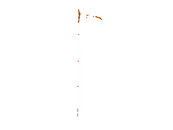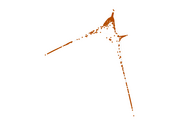### Edge weight/multiplicity distribution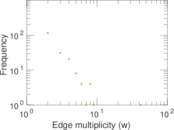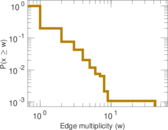### Temporal distribution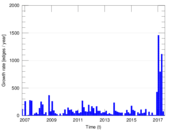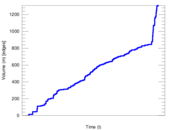### Temporal hop distribution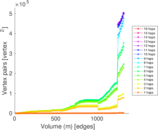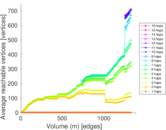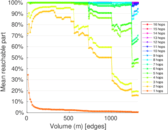### Diameter/density evolution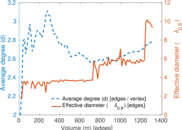### Matrix decompositions plots